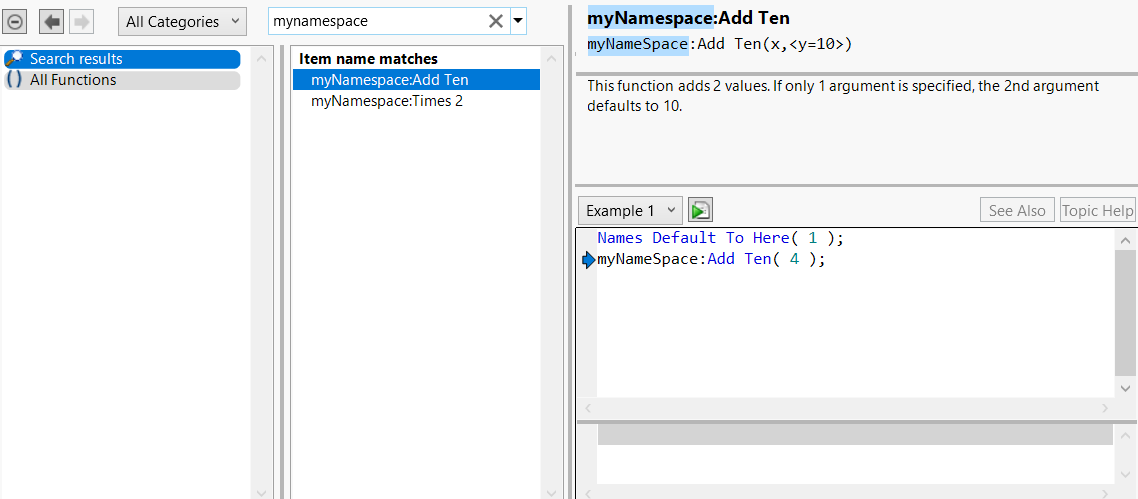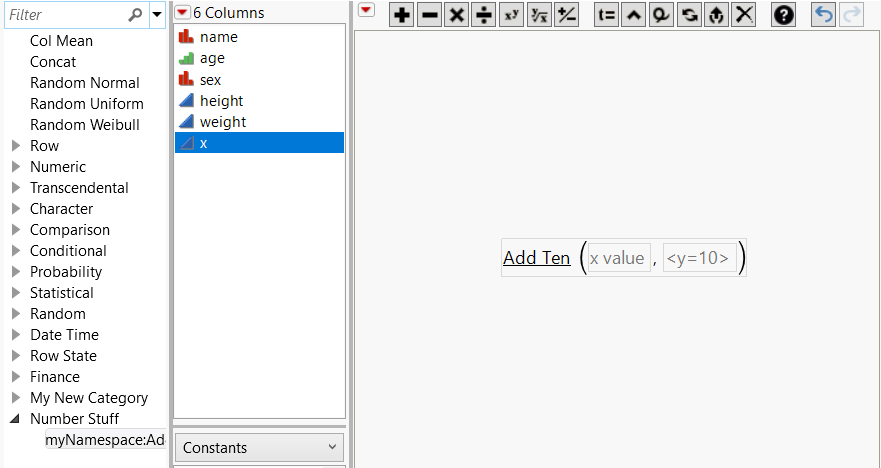Scripting Guide > Data Tables > Columns > Create Custom Functions, Transforms, and Formats
Publication date: 11/10/2021

## Create Custom Functions, Transforms, and Formats

You can create user-defined custom functions and then use them in the Formula Editor’s function list, in JSL scripting, as custom formats, and as custom transforms. Note that these custom functions can only be used in the context of the current JMP session. Restarting JMP removes previously defined custom functions. They must be defined and added for each session. An easy way to do this is to define your functions in an add-in or a startup script that runs each time you start JMP.

To define a custom function, use New Custom Function(). After a function is defined, you can add or activate it in the current running instance of JMP using Add Custom Functions(). Similarly, you can remove or deactivate a function using Remove Custom Functions().

New Custom Function() requires three parameters: namespace, functionName, functionDefinition. Additional messages may be passed to define the following:

Formula Editor values: Formula category, Result type, Parameters

Custom transform values: Transform category

Custom format values: Custom format category

Scripting Index values: Description, Prototype, Scripting Index category, Examples

Tip: If you receive a data table that uses a custom transform, function, or format, you’ll see "###" in the column. The data table author must give you a script to add that custom item to your computer. Run the script and then reopen the data table.

### Custom Function Example

Let’s create a completely specified function (including Scripting Index information and hint text). We will define the function first, then activate it.

`funcDef = Function( {x, y = 10}, x + y );`
`description = "This function adds 2 values. If only 1 argument is specified, the 2nd argument defaults to 10.";`
` `

// examples can be multiple statements inside expr()

`ex2 = Expr(`
`	dt = Open( "\$SAMPLE_DATA/Big Class.jmp" );`
`	myNameSpace:Add Ten( :age, 20 );`
`);`
`parmHint1 = "x value";`
`parmHint2 = "<y=10>";`
` `

/* create function using variables

the commands can also be accepted as arguments in New Custom Function */

`newAdd = New Custom Function( "myNamespace", "Add Ten", funcDef );`
`newAdd << Description( description );`
`newAdd << Prototype( "myNameSpace:Add Ten(x,<y=10>)" );`
`newAdd << Example( "myNameSpace:Add Ten(4)" );`
` `

// expr() Examples need to be passed inside NameExpr()

`newAdd << Example( Name Expr( ex2 ) );`
`newAdd << Parameter( "Number", parmHint1 );`
`newAdd << Parameter( "Number", parmHint2 );`
`newAdd << Formula Category( "NumberStuff" );`
` `

`Add Custom Functions( newAdd );`

Running the above example will write “Deploying function: myNamespace:Add Ten” in the JMP log. Search for this function in the Scripting Index to see the results of using the Description, Prototype, and Example messages.

Figure 9.13 Custom Function in the Scripting IndexThe Formula Category message results in a new category in the Formula Editor’s list of formula categories. Custom functions are shown with an underline in the Formula Editor. Also see the hint text from the Parameter messages.

Figure 9.14 Custom Function in the Formula Editor### Delete a Custom Function Example

To delete a custom function, use Remove Custom Functions( {"name"} ).

`Remove Custom Functions( {"myNamespace:Add Ten"} );`